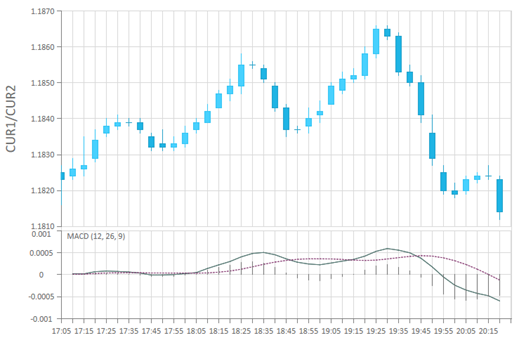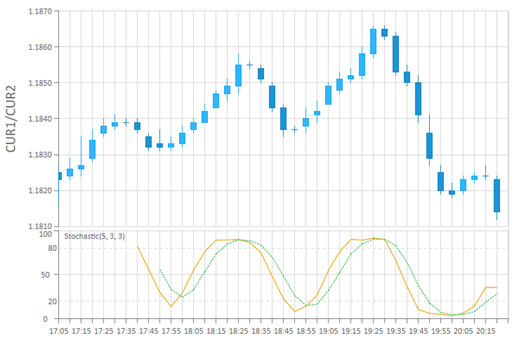# Stock Chart (Signals and Technical Indicators)BCGControlBar Pro (MFC)BCGSuite (MFC)BCGControlBar for .NET

The following chart formulas are implementing stock charts with signals and technical indicators:

• CBCGPChartRSIFormula. Implements RSI financial indicator.
• CBCGPChartMACDFormula. The formula is displayed as a histogram series and represents difference between slow and fast EMA. To show signal line you need to create an MA formula for MACD series.
• CBCGPChartStochasticFormula. Implements Stochastic Oscillator.

#### RSI (Relative Strength Index) formula:#### MACD (Moving Average Convergence/Divergence Oscillator) formula:#### Stochastic indicator: